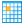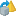You are viewing the RapidMiner Studio documentation for version 9.5 - Check here for latest version

#Normalize (RapidMiner Studio Core)

## Synopsis

This Operator normalizes the values of the selected Attributes.

## Description

Normalization is used to scale values so they fit in a specific range. Adjusting the value range is very important when dealing with Attributes of different units and scales. For example, when using the Euclidean distance all Attributes should have the same scale for a fair comparison. Normalization is useful to compare Attributes that vary in size. This Operator performs normalization of the selected Attributes. Four normalization methods are provided. These methods are explained in the parameters.

## Differentiation

###Scale by Weights

This Operator can be used to scale Attributes by pre-calculated weights. Instead of adjusting the value range to a common scale, this Operator can be used to give important Attributes even more weight.

###De-Normalize

This Operator can be used to revert a previously applied normalization. It requires the preprocessing model returned by a Normalization Operator.

## Input

•example set (IOObject)

This input port expects an ExampleSet.

## Output

•example set (IOObject)

The ExampleSet with the selected Attributes in normalized form is output of this port.

•original (IOObject)

The ExampleSet that was given as input is passed through without changes.

•preprocessing model (Preprocessing Model)

This port delivers the preprocessing model. It can be used by the Apply Model Operator to perform the specified normalization on another ExampleSet. This is helpful for example if the normalization is used during training and the same transformation has to be applied on test or actual data. The preprocessing model can also be grouped together with other preprocessing models and learning models by the Group Models Operator.

## Parameters

• create_view

Create a View instead of changing the underlying data. If this option is checked, the normalization is delayed until the transformations are needed. This parameter can be considered a legacy option.

Range:
• attribute_filter_type

This parameter allows you to select the Attribute selection filter; the method you want to use for selecting Attributes. It has the following options:

• all: This option selects all the Attributes of the ExampleSet, so that no Attributes are removed. This is the default option.
• single: This option allows the selection of a single Attribute. The required Attribute is selected by the attribute parameter.
• subset: This option allows the selection of multiple Attributes through a list (see parameter attributes). If the meta data of the ExampleSet is known, all Attributes are present in the list and the required ones can easily be selected.
• regular_expression: This option allows you to specify a regular expression for the Attribute selection. The regular expression filter is configured by the parameters regular expression, use except expression and except expression.
• value_type: This option allows selection of all the Attributes of a particular type. It should be noted that types are hierarchical. For example, both real and integer types belong to the numeric type. The value type filter is configured by the parameters value type, use value type exception, except value type.
• block_type: This option allows the selection of all the Attributes of a particular block type. It should be noted that block types may be hierarchical. For example, value_series_start and value_series_end block types both belong to the value_series block type. The block type filter is configured by the parameters block type, use block type exception, except block type.
• no_missing_values: This option selects all Attributes of the ExampleSet, which do not contain a missing value in any Example. Attributes that have even a single missing value are removed.
• numeric_value_filter: All numeric Attributes whose Examples all match a given numeric condition are selected. The condition is specified by the numeric condition parameter. Please note that all nominal Attributes are also selected irrespective of the given numerical condition.
Range:
• attribute

The required Attribute can be selected from this option. The Attribute name can be selected from the drop down box of the parameter if the meta data is known.

Range:
• attributes

The required Attributes can be selected from this option. This opens a new window with two lists. All Attributes are present in the left list. They can be shifted to the right list, which is the list of selected Attributes that will make it to the output port.

Range:
• regular_expression

Attributes whose names match this expression will be selected. The expression can be specified through the edit and preview regular expression menu. This menu gives a good idea of regular expressions and it also allows you to try different expressions and preview the results simultaneously.

Range:
• use_except_expression

If enabled, an exception to the first regular expression can be specified. This exception is specified by the except regular expression parameter.

Range:
• except_regular_expression

This option allows you to specify a regular expression. Attributes matching this expression will be filtered out even if they match the first expression (expression that was specified in regular expression parameter).

Range:
• value_type

This option allows to select a type of Attribute. One of the following types can be chosen: nominal, numeric, integer, real, text, binominal, polynominal, file_path, date_time, date, time.

Range:
• use_value_type_exception

If enabled, an exception to the selected type can be specified. This exception is specified by the except value type parameter.

Range:
• except_value_type

The Attributes matching this type will be removed from the final output even if they matched the type selected before, specified by the value type parameter. One of the following types can be selected here: nominal, numeric, integer, real, text, binominal, polynominal, file_path, date_time, date and time.

Range:
• block_type

This option allows to select a block type of Attribute. One of the following types can be chosen: single_value, value_series, value_series_start, value_series_end, value_matrix, value_matrix_start, value_matrix_end and value_matrix_row_start.

Range:
• use_block_type_exception

If enabled, an exception to the selected block type can be specified. This exception is specified by the except block type parameter.

Range:
• except_block_type

The Attributes matching this block type will be removed from the final output even if they matched the type selected before by the block type parameter. One of the following block types can be selected here: single_value, value_series, value_series_start, value_series_end, value_matrix, value_matrix_start, value_matrix_end and value_matrix_row_start.

Range:
• numeric_condition

The numeric condition used by the numeric condition filter type. A numeric Attribute is kept if all Examples match the specified condition for this Attribute. For example, the numeric condition '> 6' will keep all numeric Attributes having a value of greater than 6 in every Example. A combination of conditions is possible: '> 6 && < 11' or '<= 5 || < 0'. But && and || cannot be used together in one numeric condition. Conditions like '(> 0 && < 2) || (>10 && < 12)' are not allowed because they use both && and ||. Nominal Attributes are always kept, regardless of the specified numeric condition.

Range:
• invert_selection

If this parameter is set to true, the selection is reversed. In this case, all Attributes matching the specified condition are removed and the other Attributes remain in the output ExampleSet. Special Attributes are kept independent of the invert selection parameter as along as the include special attributes parameter is not set to true. If so, the condition is also applied to the special Attributes and the selection is reversed if this parameter is checked.

Range:
• include_special_attributes

Special Attributes are Attributes with special roles. These are: id, label, prediction, cluster, weight and batch. Also custom roles can be assigned to Attributes. By default, all special Attributes are delivered to the output port irrespective of the conditions in the Select Attributes Operator. If this parameter is set to true, special Attributes are also tested against conditions specified in the Select Attributes Operator and only those Attributes are selected that match the conditions.

Range:
• method

Four methods are provided here for normalizing data. These methods are also explained in the attached tutorial Process.

• z_transformation: This is also called statistical normalization. This normalization subtracts the mean of the data from all values and then divides them by the standard deviation. Afterwards, the distribution of the data has a mean of zero and a variance of one. This is a common and very useful normalization technique. It preserves the original distribution of the data and is less influenced by outliers.
• range_transformation: Range transformation normalizes all Attribute values to a specified value range. When this method is selected, two other parameters (min, max) appear in the Parameters panel. So the largest value is set to 'max' and the smallest value is set to 'min'. All other values are scaled, so they fit into the given range. This method can be influenced by outliers, because the bounds move towards them. On the other hand, this method keeps the original distribution of the data points, so it can also be used for data anonymization, for example to obfuscate the true range of observations.
• proportion_transformation: This normalization is based on the proportion each Attribute value has on the complete Attribute. This means each value is divided by the total sum of that Attribute values. The sum is only formed from finite values, ignoring NaN/missing values as well as positive and negative infinity. When this method is selected, another parameter (allow negative values) appears in the Parameters panel. If checked, negative values will be treated as absolute values, otherwise they will produce an error when executed.
• interquartile_range: Normalization is performed using the interquartile range. The interquartile range is the distance between the 25th and 75th percentile, which are also called lower and upper quartile, or Q1 and Q3. They are calculated by first sorting the data and then taking the data value that separates the first (or the last) 25% of the Examples from the rest. The median is the 50th percentile, so it is the value that separates the sorted values in half. The interquartile range (IQR) is the difference between Q3 and Q1. The final formula for the interquartile range normalization is then: (value median) / IQR The IQR is the range between the middle 50% of the data, so this normalization method is less influenced by outliers. NaN/missing values, as well as infinite values will be ignored for this method. Also, if no finite values could be found, the corresponding Attribute will be ignored.
Range:
• min

This parameter is available only when the method parameter is set to 'range transformation'. It is used to specify the minimum point of the range.

Range:
• max

This parameter is available only when the method parameter is set to 'range transformation'. It is used to specify the maximum point of the range.

Range:
• allow_negative_values

This parameter is available only when the method parameter is set to 'proportion transformation'. It is used to allow or disallow negative values in the processed Attributes. Negative values then will be counted as their absolute values.

Range:

## Tutorial Processes

### Normalizing Age and Passenger Fare for the Titanic data

This tutorial Process takes the Age and the Passenger Fare Attributes from the Titanic data and performs a normalization on them. The Attributes have a very different range of values (the highest Age is 80 and the highest fare is around 500). Also, the Passenger Fare has one value that is much higher than all the other fares. So it can be considered as an outlier. When applying the Z-Transformation, both Attributes are centered around 0. When changing the method to Interquartile Range, the values of the Passenger Fare are spread out a bit more evenly, as the one outlier does not have so much influence. For visualization, it is best to use the Histogram charts view.